# Dijkstra's Algorithm in Java | Find Shortest Path (with full code)

Have you ever wondered how google maps always find out the shortest path between any two locations for us? Which algorithm does it use? Does it implement Dijkstra's Algorithm? You will be able to answer these questions and learn how to implement Dijkstra's Algorithm in Java till you reach the end of this article.

But before we deep dive into this topic, let's go through the basics so that we easily understand it.

### What is a Graph?

A graph is a non-linear data structure containing vertices or nodes that are connected through edges. Technically, a graph is a set of U elements (vertices/nodes) and E edges. Each edge of the graph can be represented by unordered pair [x,y] (x,y are nodes in U), generally denoted by e = [x,y].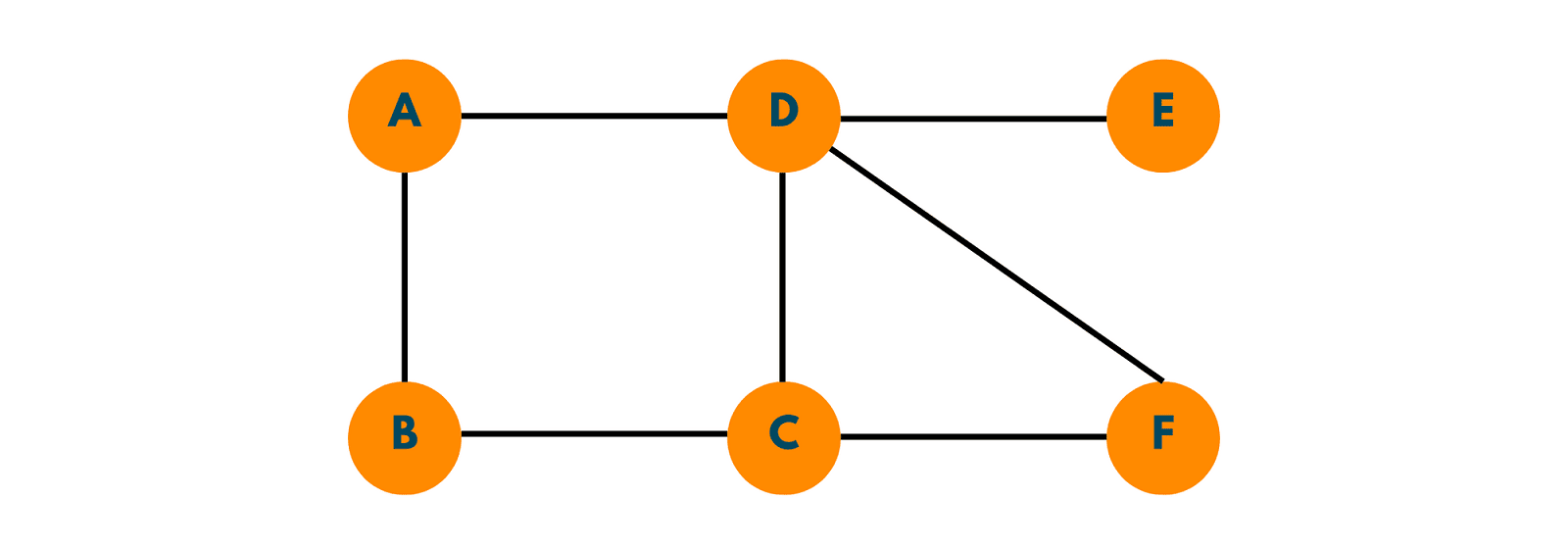Here A, B, C, D, E, and F are the vertices/nodes of the graph and the line through which these edges are connected is called the Edges of a graph.

The graph is of two types :

1. Directed Graphs: If each edge of the graph is assigned with a direction, i.e. one-way movement, is called a directed graph.
2. Undirected graph: When no direction is assigned on any of the edges of the graph i.e. one can move in any in either directed between 2 nodes, is called an undirected graph.

As no directions have been mentioned on edges, that implies it is an undirected graph. If we consider vertices A and B, one can freely move from A to B or B to A.

### What is a Weighted Graph?

Graph G is said to be a weighted graph if its edge has been assigned any non-negative value. The value assigned to the edge can be cost, time, or the distance portraying the relationship between 2 nodes connected through that edge.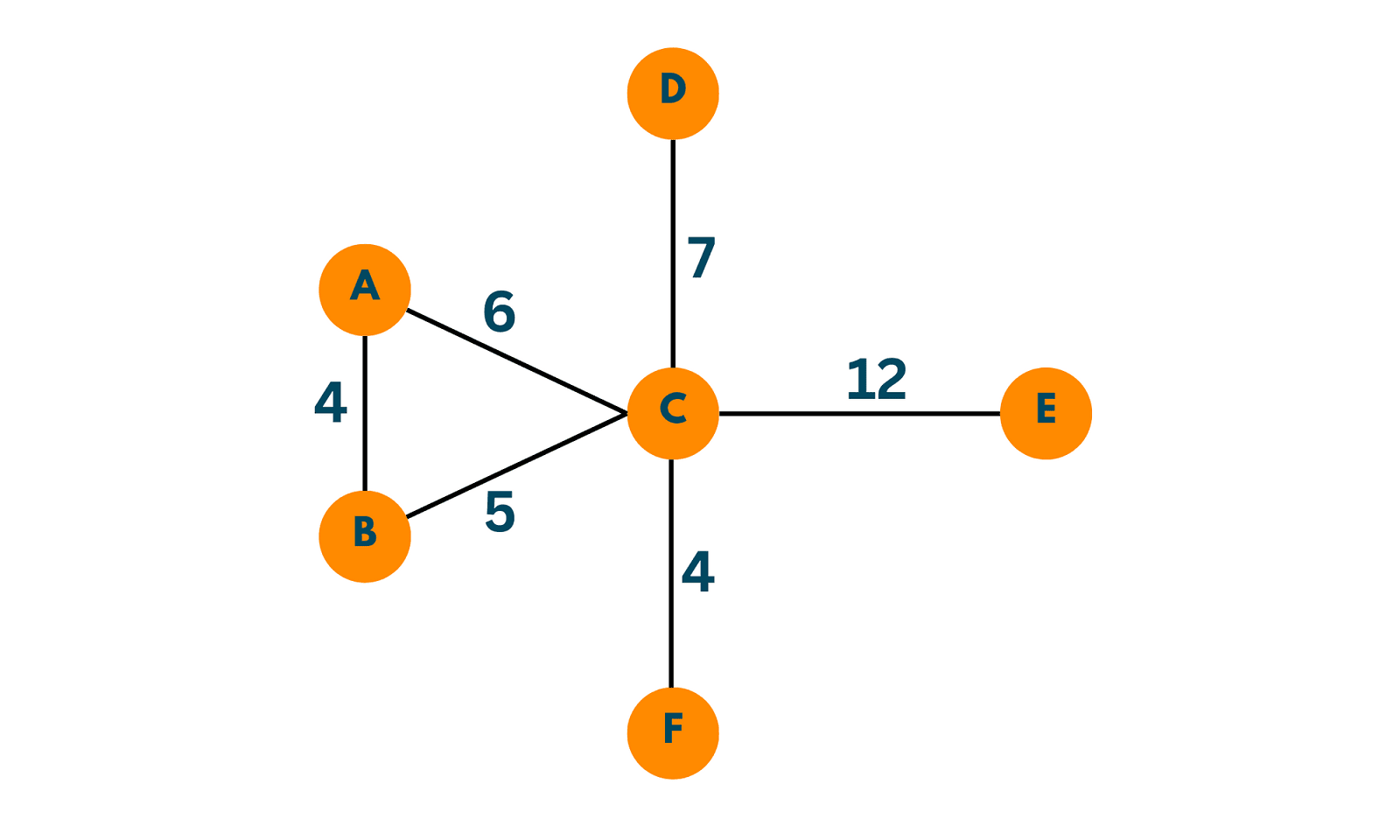As nonnegative values have been mentioned on edges it is a weighted graph. These values can be the cost to travel from one place to another or time or distance.

## What is Dijkstra's Algorithm?

Dijkstra's algorithm is used in finding the shortest path between any 2 given nodes of the graph. This algorithm only works for a weighted, undirected/directed graph and it is also called a single source shortest path problem. But it can also be used to solve multiple-source shortest path problems by simply running the algorithm for each source node. It uses a greedy Approach and always provides the optimal answer.

### Basic Architecture of the Algorithm

It starts working from the source node and calculates each distance to neighboring nodes (basically checking all the possible paths to the next neighboring node). It then takes up the path to the next node which has the lowest cost out of all possible options. It repeats the same process until it reaches the destination node.

Let's understand it through an interesting Example. Consider the above graph depicting countries and the distance between them. Imagine Juhi has to travel from India to the USA.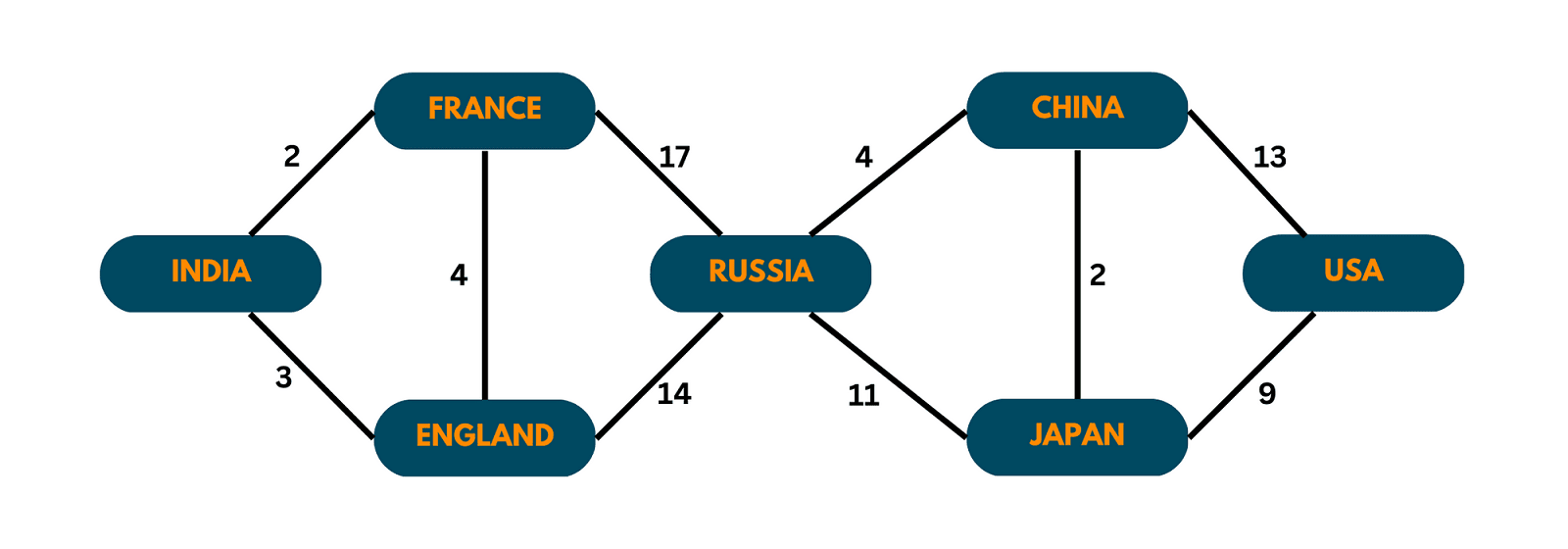So as Juhi is starting her journey from India, that implies the source node would be India. When she has to reach the USA, the destination node becomes the USA. In order to find the shortest path from India to the USA, we will use Dijkstra's Algorithm.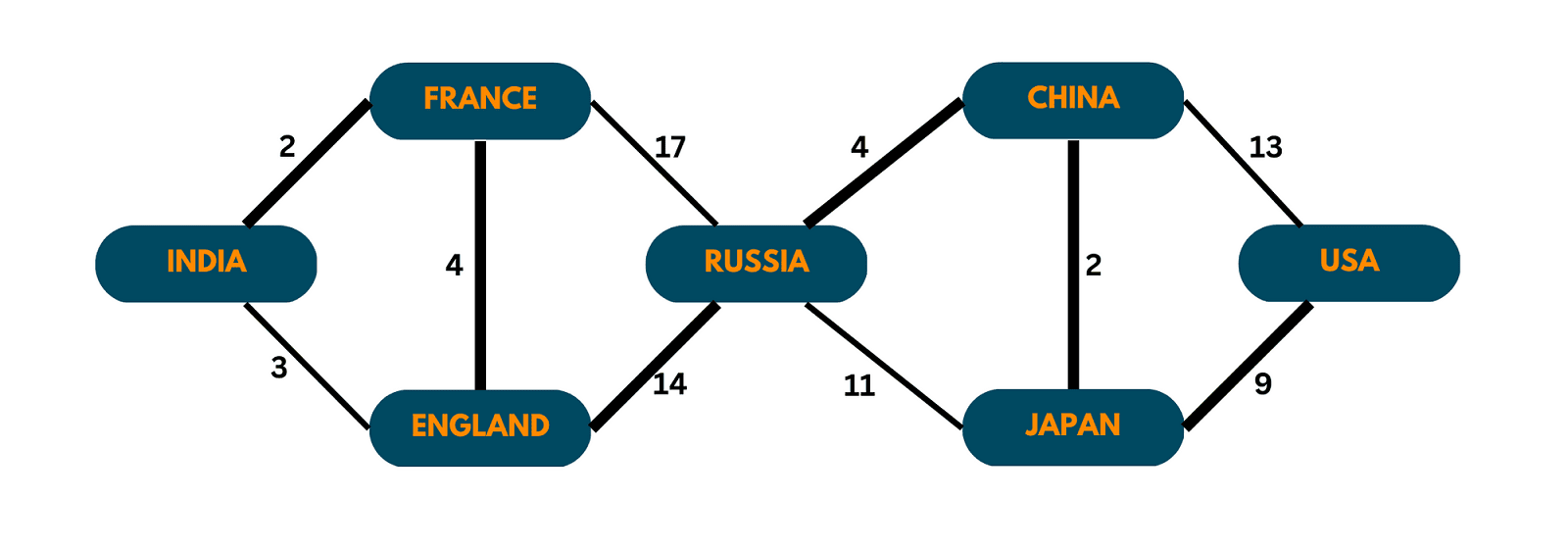This algorithm wisely takes up the edges that have less weight and discards the ones having more weight which leads us to the destination node.

Dijkstra's algorithm is used to find the shortest path between any 2 locations provided (as used in Google Maps), or in the routing algorithm. You can utilize it efficiently in transportation networks as it can help minimize travel time and fuel consumption.

Let's learn how this algorithm does so.

## Implement Dijkstra's Algorithm in Java

We have 2 methods for the implementation of Dijkstra's algorithm in Java:

### Method 1) Using Adjacency Matrix

Here is the complete approach:

1. Create a visited boolean array of size = vertices, that initially contain false at each index describing that no vertex has been visited yet.
2. Create an integer array called distance that contains the distance of that node from the source node.
3. Mark distance[source] = 0 and the distance of other vertices to be infinity. Also, mark visited [source] = true.
4. Start from the source node and explore all the neighbors of the source node that have been marked as non-visited.
5. If the weight of edge + distance [currentVertex] is less than distance[vertex], then update the distance[vertex] = weight of Egde + distance[currentVertex].
6. Repeat step 5 for all the unvisited neighbors of the current vertex.
7. Now we take the index having a minimum value from the distance array whose visited is marked as false as the next current vertex.
8. Repeat until all vertices have been marked visited.

Below we have given the full code in Java to implement  Dijkstra's algorithm using the adjacency matrix:

```import java.io.*;
import java.util.*;
public class Main {

public static void main(String[] args) {
int adjMat [] [] = {
{0, 2, 0, 4, 0, 0},
{0, 0, 3, 2, 0, 0},
{2, 0, 0, 0, 0, 4},
{0, 0, 0, 0, 2, 0},
{0, 0, 0, 0, 0, 1},
{0, 0, 0, 0, 0, 0}};

//creating a distance array to keep a note of distance of vertex from source
int [] distance = new int[adjMat.length];

//taking source vertex to be 0
int source = 0;

//creating a visited array to keep a count of visited vertices.
boolean [] visited = new boolean[adjMat.length];

//marking distance of source vertex
distance[source] = 0;

// filling up other distance in array "distance" as infinity or the max value
for (int i = 0; i < adjMat.length; i++) {
if( i == source) continue;
distance[i] = Integer.MAX_VALUE;
}

//finding the vertex that is most close to current node or source
for(int i = 0; i < adjMat.length; i++) {
int minDistVertex = findMinDistVertex(distance, visited);

//marking the vertex that is most close to source/current vertex as true
visited[minDistVertex] = true;

//exploring the neighbors of each vertex and updating distance array with new distance
for(int j = 0; j < adjMat.length; j++) {
if(adjMat[minDistVertex][j] != 0 && visited[j] == false && distance[minDistVertex] != Integer.MAX_VALUE) {
int newDist = distance[minDistVertex] + adjMat[minDistVertex][j];
if(newDist < distance[j]) {
distance[j] = newDist;
}
}
}
}

for(int i = 0; i < adjMat.length; i++) {
System.out.println("Vertex : " + i + " & Distance from Source : " +distance[i]);
}
}

public static int findMinDistVertex(int[] distance, boolean [] visited) {

int minVertex = -1;

//traversing through the distance array and finding the least distance vertex whose visited is also false
for(int i = 0; i < distance.length; i++) {
if(visited[i] == false && (minVertex == -1 || distance[i] < distance[minVertex])) {
minVertex = i;
}
}
return minVertex;
}
}
```

Output:

```Vertex : 0 & Distance from Source : 0
Vertex : 1 & Distance from Source : 2
Vertex : 2 & Distance from Source : 5
Vertex : 3 & Distance from Source : 4
Vertex : 4 & Distance from Source : 6
Vertex : 5 & Distance from Source : 7
```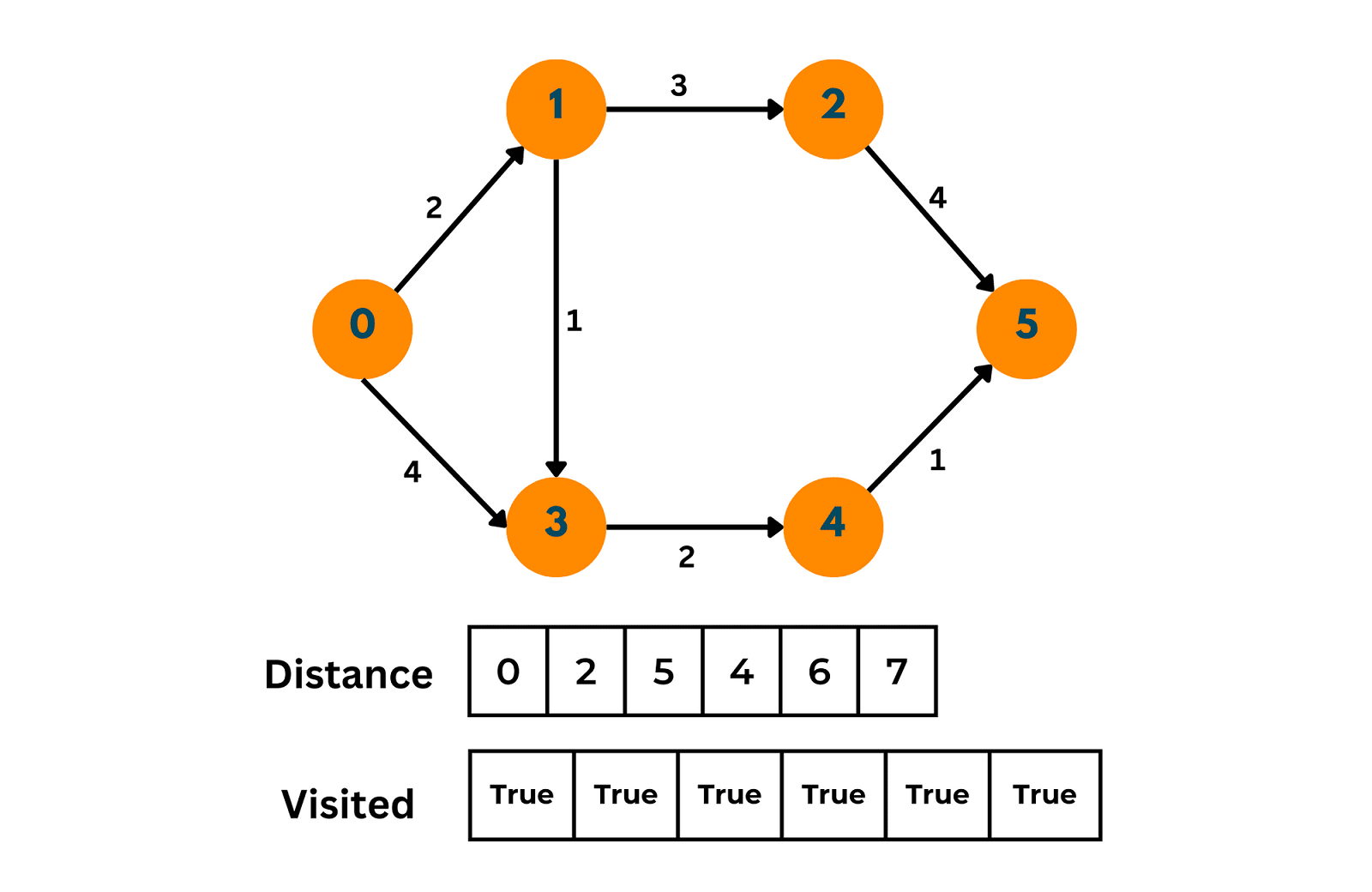### Method 2) Using Priority Queue

Here is the complete approach:

1. Create a visited boolean array of size = no.of vertices, where each index of the array points to each vertex.
2. Initially, all values in the array are false, depicting no vertex has been visited.
3. Then we make a priority queue PrQue, storing objects of type Pair. A pair consisting of 2 data members: vertex and weight to reach that vertex(w).
4. The priority queue arranges the objects in terms of the weight to reach that vertex(w).
5. Initially we insert a Pair(sourceVertex, 0) in the priority Queue.
6. Now till PrioQue is not empty  we follow the procedure -> remove, mark, print, add i.e
1. Remove the topmost element from the priority queue and store it in a variable say topEle.
2. Mark the topmost element as visited in the boolean array. If it is already visited then continue.
3. Print the vertex value and the weight so far.
4. Now add all the neighbors of topEle to the priority queue only and only if they have not been visited before.
7.  Exit.

Below we have given the full code in Java to implement  Dijkstra's algorithm using the Priority Queue:

```import java.io.*;
import java.util.*;
public class Main {

// making an edge class that stores the vertex and its neighbor along with the weight
static class Edge {
int source;
int nbr;
int weight;

Edge(int source, int nbr, int weight) {
this.source = source;
this.nbr = nbr;
this.weight = weight;
}
}

//making a pair class
static class Pair implements Comparable< Pair> {
int vertex;
int wsf; //weight so far

Pair(int vertex, int wsf) {
this.vertex = vertex;
this.wsf = wsf;
}
// using CompareTo function so that priority queue arranges the element in terms of weight so far
public int compareTo(Pair o) {
return this.wsf - o.wsf;
}
}

public static void main(String[] args) {

// building the graph
// the graph is an array of ArrayList (of Object,Edge)
// length of array = the vertices present in graph.

ArrayList<Edge> [] graph =  new ArrayList;
for(int i = 0; i < graph.length; i++){
graph[i] = new ArrayList<>();
}

// Adding all the vertices and its members along with weights

//taking 0 as source node
int src = 0;

//creating a visited boolean array
boolean[] visited = new boolean[graph.length];

//Initialising a priority Queue of object Pair
PriorityQueue< Pair> prioQue = new PriorityQueue<>();

// while queue is not empty following the procedure remove,mark,print,add

while (prioQue.size() > 0) {

// removing the topmost element and storing it in topEle
Pair topEle = prioQue.remove();

if (visited[topEle.vertex] == true) {
continue;
}
// marking true if  vertex not visited
visited[topEle.vertex] = true;

//Printing the vertex and weight to reach that vertex
System.out.println("Vertex :" + " " +topEle.vertex + " & " + "Weight so far :" + " " +topEle.wsf);

//adding all the unvisited neighbor of vertex in queue
for (Edge edge : graph[topEle.vertex]) {
if (visited[edge.nbr] == false) {
prioQue.add(new Pair(edge.nbr, topEle.wsf + edge.weight ));
}
}
}
}
}
```

Output:

```Vertex : 0 & Weight so far : 0
Vertex : 3 & Weight so far : 5
Vertex : 4 & Weight so far : 8
Vertex : 1 & Weight so far : 10
Vertex : 2 & Weight so far : 16
Vertex : 5 & Weight so far : 17
Vertex : 6 & Weight so far : 23
```

Now this method and code would work for vertices having values from 0 to 6 i.e. till number of vertices -1. If the value of vertices would have been a string or any other number, then we would have used a hashmap where the key would vary from 0 to no.of vertices-1, and the value of the hashmap would be the value of vertices.

Instead of a hashmap, we can also use an array where the indices of the array can be treated as a key and the vertex value can be treated as an array[index].

For example: If the graph consisted of countries' names instead of numbers then, check the new code before:

```package Graphs;
import java.io.*;
import java.util.*;
public class Main {

// making an edge class that stores the vertex and its neighbor along with the weight
static class Edge {
int source;
int nbr;
int weight;

Edge(int source, int nbr, int weight) {
this.source = source;
this.nbr = nbr;
this.weight = weight;
}
}

//making a pair class
static class Pair implements Comparable< Pair> {
int vertex;
int wsf; //weight so far

Pair(int vertex, int wsf) {
this.vertex = vertex;
this.wsf = wsf;
}
// using CompareTo function so that priority queue arranges the element in terms of weight so far
public int compareTo(Pair o) {
return this.wsf - o.wsf;
}
}

public static void main(String[] args) {

// building the graph
// the graph is an array of ArrayList (of Object,Edge)
// length of array = the vertices present in graph.

ArrayList<Edge> [] graph =  new ArrayList;
for(int i = 0; i < graph.length; i++){
graph[i] = new ArrayList<>();
}

//creating a hashmap to store mapped values
HashMap<Integer, String> map= new HashMap<>();

// if graph vertices consisted of strings rather than numbers
map.put(0, "India");
map.put(1, "China");
map.put(2, "France");
map.put(3, "England");
map.put(4, "Japan");
map.put(5, "America");
map.put(6, "Russia");

// Adding all the vertices and its members along with weights

//taking 0 as source node
int src = 0;

//creating a visited boolean array
boolean[] visited = new boolean[graph.length];

//Initialising a priority Queue of object Pair
PriorityQueue< Pair> prioQue = new PriorityQueue<>();

// while queue is not empty following the procedure remove,mark,print,add

while (prioQue.size() > 0) {

// removing the topmost element and storing it in topEle
Pair topEle = prioQue.remove();

if (visited[topEle.vertex] == true) {
continue;
}
// marking true if  vertex not visited
visited[topEle.vertex] = true;

//Printing the vertex and weight to reach that vertex
System.out.println("Vertex :" + " " +map.get(topEle.vertex) + " & " + "Weight so far :" + " " +topEle.wsf);

//adding all the unvisited neighbor of vertex in queue
for (Edge edge : graph[topEle.vertex]) {
if (visited[edge.nbr] == false) {
prioQue.add(new Pair(edge.nbr, topEle.wsf + edge.weight ));
}
}
}
}
}
```

Output:

```Vertex : India & Weight so far : 0
Vertex : England & Weight so far : 5
Vertex : Japan & Weight so far : 8
Vertex : China & Weight so far : 10
Vertex : France & Weight so far : 16
Vertex : America & Weight so far : 17
Vertex : Russia & Weight so far : 23
```

### Is Djikstra's Algorithm BFS?

Dijkstra's algorithm is a priority first algorithm. We can say that, Dijkstra's is a generalization of BFS, not BFS exactly, but a generalization. As we know a queue is used in the BFS algorithm but while implementing Dijkstra's algorithm, we use a priority queue.

Dijkstra's algorithm is described as a generalized form of BFS, here the order of traversed nodes doesn't depend on the number of edges from the source vertex but on the distance from the source vertex. There is also a difference between the time complexities of BFS and Dijkstra i.e. O(E+V)  and O((E + VlogV)) respectively where E is the edge and V is the vertex of the graph.

### Is Dijkstra's Algorithm greedy or dynamic programming?

Dijkstra is a greedy method because it chooses a local optimum at each step. Dijkstra always gives the shortest path when the marginal costs are all positive. However, this can fail when the edge costs are negative. While the dynamic programming solution always gives the shortest path, even if the marginal cost is negative.

So this is generally considered a greedy algorithm because it basically uses a greedy selection property to get the optimal value.

### Time Complexity Analysis

The time complexity of Dijkstra's algorithm is O((E + VlogV)), where V is no of vertices and E is no. of edges of the graph. We iterate over all the edges only once during the whole execution of the algorithm because at every iteration we pop out an edge and we add only those neighbors of that vertex that aren't visited yet.

As the priority queue can contain all the edges at some time during the execution, so add/removing time complexity would be O(log V). The maximum size of the priority queue can be O(E). Therefore total time complexity becomes O(E) + O(V) *O(log V).

## Conclusion

Overall, Dijkstra's algorithm is a powerful tool that can be used to solve optimization problems in various fields. This algorithm has countless applications which can be represented as graphs. You can now implement Dijkstra's Algorithm in Java using the 2 main approaches.

### FavTutor - 24x7 Live Coding Help from Expert Tutors!